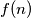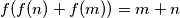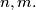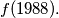### IMO Shortlist 1988 problem 19

Kvaliteta:
Avg: 2,0
Težina:
Avg: 4,0
Let$f(n)$ be a function defined on the set of all positive integers and having its values in the same set. Suppose that$f(f(n) + f(m)) = m + n$ for all positive integers$n,m.$ Find the possible value for$f(1988).$
Izvor: Međunarodna matematička olimpijada, shortlist 1988